Courses

# Test: Properties Of Isosceles Triangle

## 10 Questions MCQ Test Mathematics (Maths) Class 9 | Test: Properties Of Isosceles Triangle

Description
This mock test of Test: Properties Of Isosceles Triangle for Class 9 helps you for every Class 9 entrance exam. This contains 10 Multiple Choice Questions for Class 9 Test: Properties Of Isosceles Triangle (mcq) to study with solutions a complete question bank. The solved questions answers in this Test: Properties Of Isosceles Triangle quiz give you a good mix of easy questions and tough questions. Class 9 students definitely take this Test: Properties Of Isosceles Triangle exercise for a better result in the exam. You can find other Test: Properties Of Isosceles Triangle extra questions, long questions & short questions for Class 9 on EduRev as well by searching above.
QUESTION: 1

### In two right triangles, one side and an acute angle of one are equal to the corresponding side and angle of the other, then ΔABC ≅ ΔDEF by the criterion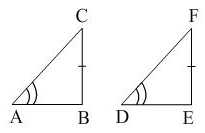Solution: Answer is B because in this it is given that angle A = D . Then also B=E as they are right angle. And finally line CB = line FE (given) there for the criteria is AAS.
QUESTION: 2

### In ΔABC if ∠A = ∠B, then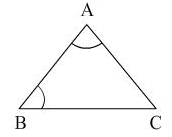Solution: Becuase Sides opposite to equal angles are also equal.angle A = angle BBC is opposite to angle A and AC is opposite to angle B.Therefore, AC=BC in triangle ABC.
QUESTION: 3

### In the given figure, AB = AC and ∠ACD = 105°, ∠BAC will be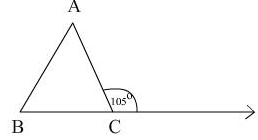Solution: Angle acb = 75 ( linear pair) Ab= Ac hence angle B= angle c in triangle ABC angle a+B+c= 180. angle B+ c = 150 hence angle c a= 30
QUESTION: 4

PQR is a triangle in which PQ = PR and S is any point on the side PQ. Through S, a line is drawn parallel to QR and intersecting PR at T. then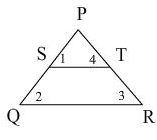Solution:
QUESTION: 5

The altitude of an equilateral triangle of side a to any of its other sides from the opposite vertex is

Solution:
QUESTION: 6

In an isosceles right angled triangle, the measures of the acute angles are​

Solution:
QUESTION: 7

In ΔABC if AB = BC then:

Solution:
QUESTION: 8

Pick out the incorrect statement

Solution:
QUESTION: 9

If the bisector of the exterior vertical angle of a triangle is parallel to the base, then it is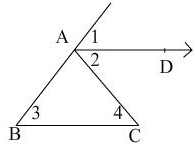Solution:
∠CAE is the external vertical angle and AD is its bisector.

∴ ∠CAD = ∠DAE  .... (i)

∴ ∠CAD = ∠ACB  .... (ii)  (alternate angle)

and ∠DAE = ∠ABC  ....(iii)  (corresponding angle)

From (i), (ii) and (iii),

∠ABC = ∠ACB

∴ AC = AB  (opposite sides to equal angles)

Hence, ΔABC is isosceles.
QUESTION: 10

In PQR, PQ = PR and R = 65°, then P = ?

Solution:

By using the theorem,If two sides of a triangle are equal then the opposite angles to the sides are equal.
⇒ if PQ=PR then ∠Q=∠R
in triangle PQR,
⇒ ∠P+∠Q+∠R=180°
⇒ ∠P+∠Q+∠Q=180° (∵∠Q=∠R)
⇒ ∠P+65°+65°=180°
⇒ ∠P+130°=180°
⇒ ∠P=180°-130°
⇒ ∠P=50